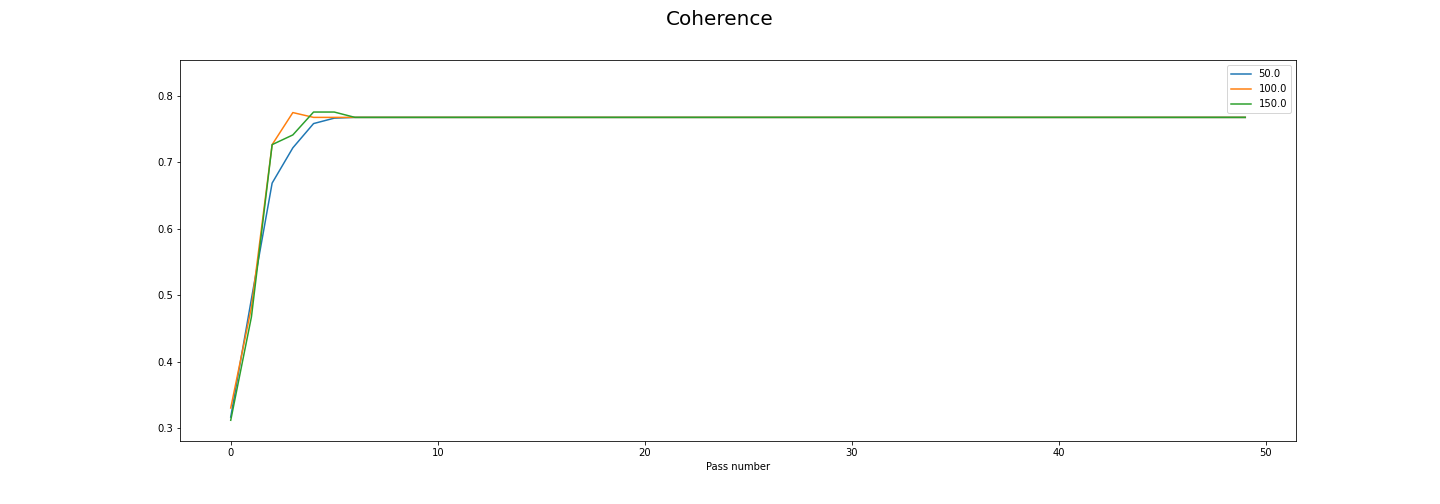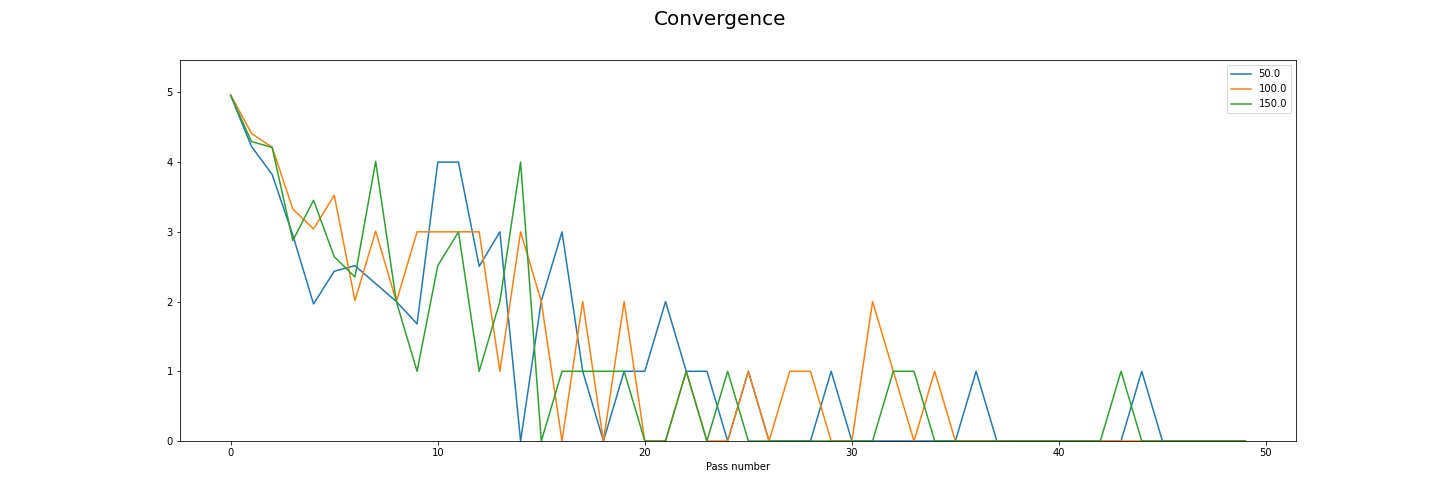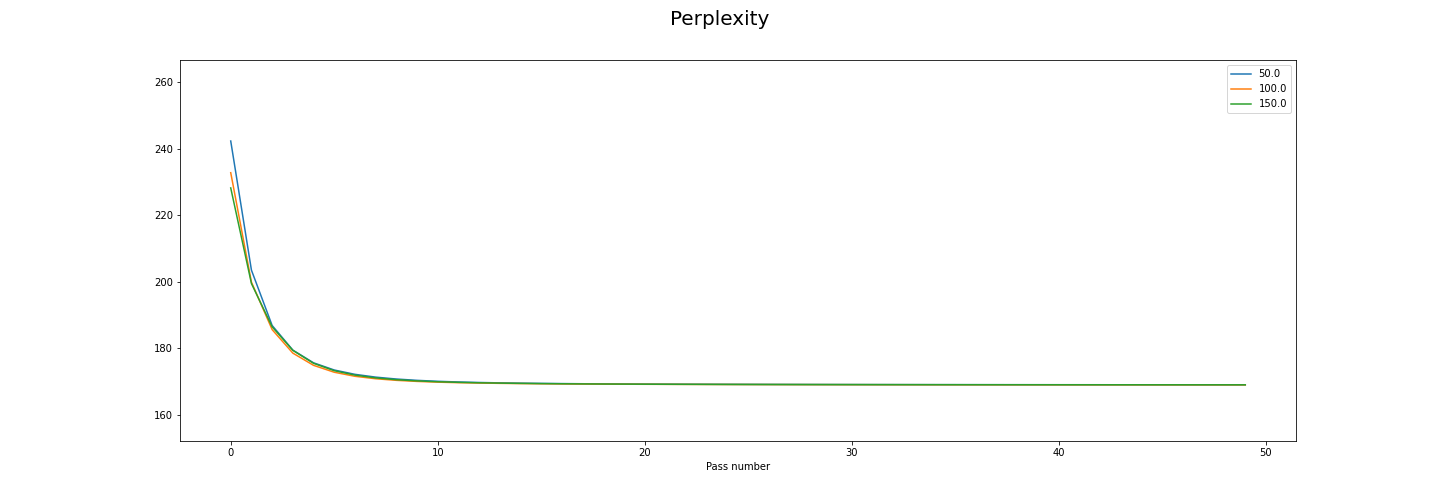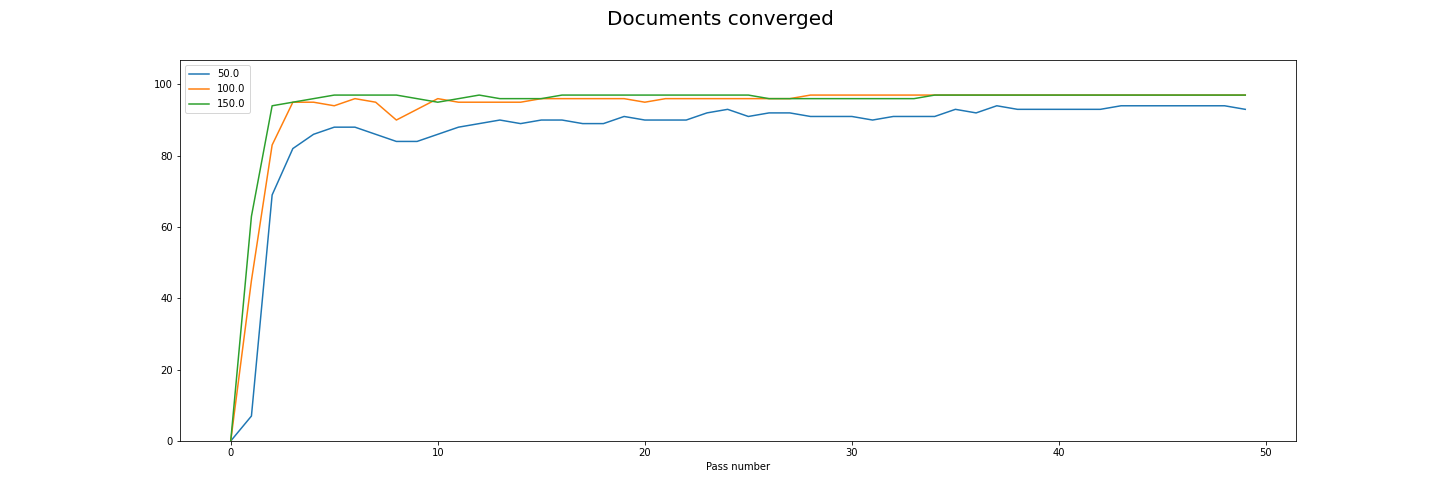# Using callbacks and logging during training with gensim

How long should you train an LDA model for? This post is less to do with the actual minutes and hours it takes to train a model, which is impacted in several ways, but more do with the number of opportunities the model has during training to learn from the data, and therefore the ultimate quality of the model. Using the python package `gensim` to train an LDA model, there are two hyperparameters in particular to consider.

The first one, `passes`, relates to the number of times the model is trained on the entire corpus. The default value in `gensim` is 1, which will sometimes be enough if you have a very large corpus, but often benefits from being higher to allow more documents to converge.

Secondly, `iterations` is more to do with how often a particular route through a document is taken during training. More technically, it controls how many iterations the variational Bayes is allowed in the E-step without convergence - so there is a cap on how long the process will run if all documents have not achieved convergence. This paper on LDA includes the algorithm of importance at the top of page 4 if you’re interested in more detail. The default is 50 in `gensim`.

There are a few ways you could choose the number of passes. You could just make them really high - but the more passes you have, the longer it will take to train your model, so you might be unnecessarily adding a lot of training time into your process. You could train a few models at different levels and use those to work out an optimal level. However, that requires you to train multiple models and still only get a few data points.

The useful thing about callbacks is that you can train one model and get the metrics you are interested in at each pass. This means you can easily chart how the metric changes as the number of passes increases. You can confirm if the metric has reached a plateau, with further passes unlikely to reap further improvements. You can also see if in fact performance is decreasing! And it’s useful to be able to find the number of passes where performance stops improving notably, because you might well be training a lot more versions of models to experiment with other things (such as topic number or preprocessing options), so you want to have the best number of passes without going unnecessarily high and dealing with long training times.

Although you can only check one value for iterations at a time, I would normally combine my attempt to select passes and iterations together, by training a few models with different iteration values and using callbacks to compare performance over passes.

Note that adding callbacks in does increase training time, so only use them when you are actually going to be reviewing them!

## Metrics to look at with callbacks

These are the metrics provided in existing `gensim` callback classes.

### Coherence

A measure of similarity between top scoring words in topics. The version used here is c_v coherence. Scores are between 0 and 1. Closer to 1 is better.

### Perplexity

Perplexity is a statistical measure giving the normalised log-likelihood of a test set held out from the training data. The figure it produces indicates the probability of the unseen data occurring given the data the model was trained on. The higher the figure, the more ‘surprising’ the new data is, so a low score suggests a model that adapts better to unseen data.

However, a 2009 study found that perplexity and human judgment are often not correlated. So depending on what we want, this might not be the best metric.

### Topic diff

This metric considers the difference between subtopics produced by the same model (in this case the model at the given number of passes). It is the mean of how unique the top words of each subtopic are compared with all the other subtopics. Where all the top words are totally unique for all subtopics, the score would be 1.

This can be quite a hygienic metric - basically you don’t want your model to do badly on this, but as long as it does well you would probably use other metrics to finalise your decision.

### Convergence

The convergence score provided by the callbacks class relates to topic convergence. In practice, this means it is the sum of the topic diff scores, so normally it isn’t necessary to run both.

To compare models with different numbers of topics, these should be normalised - for example, finding the average topic diff by dividing the convergence score by the topic number.

## Logging

If the callbacks metrics don’t provide what you need, you might be able to get at them through logging. For example, it’s interesting to know how many documents have converged each pass, because ideally you only want to stop training when a notable majority have converged. So as well as including callbacks, we’ll enable logging in the example.

## An example

In this example, I’ve downloaded text from five different novels from Project Gutenberg and split them into their chapters (an idea I’ve taken from Text Mining with R). Because it’s just an example, I’m using these books because they’ll almost certainly split into clean topics (i.e. books in this example) without needing a lot of data, and I’ll know how many topics there are in advance of training the models. If you had no idea about the number of topics you might have in your dataset, it might be worth running this a few times to get a sense of whether that drastically changes the outcome of your ongoing metrics, or even training a few models first to explore the number of topics quickly.

Here are the packages to import:

``````import pandas as pd
from gensim import models
import matplotlib.pyplot as plt
import logging
import re
import os

### choose the callbacks classes to import
from gensim.models.callbacks import PerplexityMetric, ConvergenceMetric, CoherenceMetric``````

Set up logging as well.

``````# The filename is the file that will be created with the log.
# If the file already exists, the log will continue rather than being overwritten.
logging.basicConfig(filename=model_callbacks.log',
format="%(asctime)s:%(levelname)s:%(message)s",
level=logging.NOTSET)``````

To use this code, you should have `documents`: a list of all documents, where each document is a string. Then if you don’t already have your corpus and dictionary, you can run this code to get them:

``````# Creating the term dictionary, where every unique term is assigned an index
dictionary = corpora.Dictionary(documents)

# Creating corpus using dictionary prepared above
corpus = [dictionary.doc2bow(doc) for doc in documents]``````

Set up the callbacks you want to use.

``````# Set up the callbacks loggers
perplexity_logger = PerplexityMetric(corpus=corpus, logger='shell')
convergence_logger = ConvergenceMetric(logger='shell')
coherence_cv_logger = CoherenceMetric(corpus=corpus, logger='shell', coherence = 'c_v', texts = documents)``````

Then train models - you could just train one, or you could try different iterations and/or topic numbers.

``````# List of the different iterations to try
iterations = [50, 100, 150]

# The number of passes to use - could change depending on requirements
passes = 50

for iteration in iterations:

# Add text to logger to indicate new model
logging.debug(f'Start of model: {iteration} iterations')

# Create model - note callbacks argument uses list of created callback loggers
model = models.ldamodel.LdaModel(corpus=corpus,
id2word=dictionary,
num_topics=5,
eval_every=20,
passes=passes,
iterations=iteration,
random_state=100,
callbacks=[convergence_logger, perplexity_logger, coherence_cv_logger])

# Add text to logger to indicate end of this model
logging.debug(f'End of model: {iteration} iterations')

# Save models so they aren't lost
if not os.path.exists(f"lda_{iteration}i50p/"):
os.makedirs(f"lda_{iteration}i50p/")

model.save(f"lda_{iteration}i50p/lda_{iteration}i50p.model")``````

I created this function to go through the saved logging file and pull out the number of documents converged at each pass. It could be simplified, but has some complexity due to the possibility of having multiple attempts at a model in the log, if you’ve rerun the code a few times. This will pick up on the latest time the logs captured the relevant model.

``````# Function to detect relevant numbers in log
def find_doc_convergence(topic_num, iteration, log):
# Regex to bookend log for iteration - choose last occurrence
end_slice = re.compile(f"End of model .*? {iteration} iterations")
end_matches = [end_slice.findall(l) for l in open(log)]
iteration_end = [i for i, x in enumerate(end_matches) if x]
iteration_end = iteration_end[-1]
start_slice = re.compile(f"Start of model .*? {iteration} iterations")
start_matches = [start_slice.findall(l) for l in open(log)]
start_options = [i for i, x in enumerate(start_matches) if x]
start_options = [item for item in start_options if item < iteration_end]
iteration_start = max(start_options)
iteration_bookends = [iteration_start, iteration_end]
# Regex to find documents converged figures
p = re.compile(":(\d+)\/\d")
matches = [p.findall(l) for l in open(log)]
matches = matches[iteration_bookends:iteration_bookends]
matches = [m for m in matches if len(m) > 0]
# Unlist internal lists and turn into numbers
matches = [m for sublist in matches for m in sublist]
matches = [float(m) for m in matches]
return(matches)``````

Then you can use the `model.metrics` to get the callbacks metrics for each model, and the function above to get the metrics from the log. This creates a dataframe of all the metrics across the models.

``````iterations = [50, 100, 150]

all_metrics = pd.DataFrame()

for iteration in tqdm(iterations):
df = pd.DataFrame.from_dict(model.metrics)

df['docs_converged'] = find_doc_convergence(5, iteration, "model_callbacks.log")
df['iterations'] = iteration
df['topics'] = 5

df = df.reset_index().rename(columns={'index': 'pass_num'})

all_metrics = pd.concat([all_metrics, df])``````

Finally, you can visualise the results using something like this:

``````for metric in ['Coherence', 'Perplexity', 'Convergence', 'docs_converged']:

fig, axs = plt.subplots(1, 1, figsize=(20, 7))

# Each plot to show results for all models with the same topic number
for i, topic_number in enumerate():
filtered_topics = all_metrics[all_metrics['topics'] == topic_number]
for label, df in filtered_topics.groupby(['iterations']):
print(label)
df.plot(x='pass_num', y=metric, ax=axs, label=label)

axs.set_xlabel(f"Pass number")
axs.legend()
axs.set_ylim([all_metrics[metric].min() * 0.9, all_metrics[metric].max() * 1.1])

if metric == 'docs_converged':
fig.suptitle('Documents converged', fontsize=20)
else:
fig.suptitle(metric, fontsize=20)``````

This gets you graphs like these:## Making a decision

Now that it’s done, you need to make a decision on those values. You probably want a sense of which metrics are most important to you and the minimum performance you would be happy with. However, broadly speaking when the metric evens out, you are unlikely to see much further improvement without changing something (more data, different processing), so as a rule of thumb I would look for the point at which relevant metrics flatten and use those points as your default values for further experiementation.

Looking at the graphs for the example data, you can see:

• Coherence improves sharply then flatlines after about 7 passes. No notable difference by iterations.

• Convergence is noisier, but generally decreases for the first 20 or so passes. Almost at 0 every time after 37 passes, though it might not be worth adding those extra passes for fairly small/uncertain gains.

• Perplexity is nice and flat after 5 or 6 passes. Iterations make no difference.

• Documents converged are pretty flat by 10 passes. There are 97 documents in this example (which is very low for topic modelling), so notably the 100 and 150 iteration models are basicaly seeing all documents converge, whereas 50 iterations is a bit below.

I think if you were uisng this data and decided to use 20 as your `passes` value and 100 as your `iterations` value, you’d have a good compromise between performance and training time. If you were not concerned about the convergence value you could use fewer passes, or if you were really concerned then you could use more.

There isn’t a definitive answer, but going through this step helps you to know what the trade-offs are and help idenitfy a good option for your work.